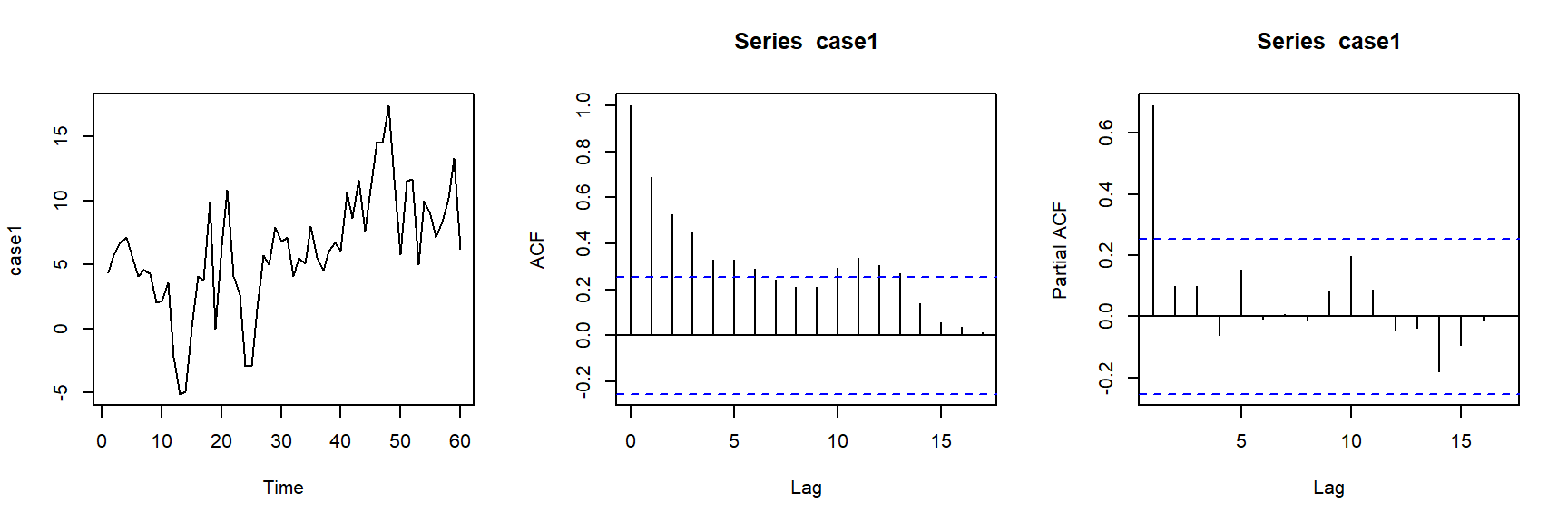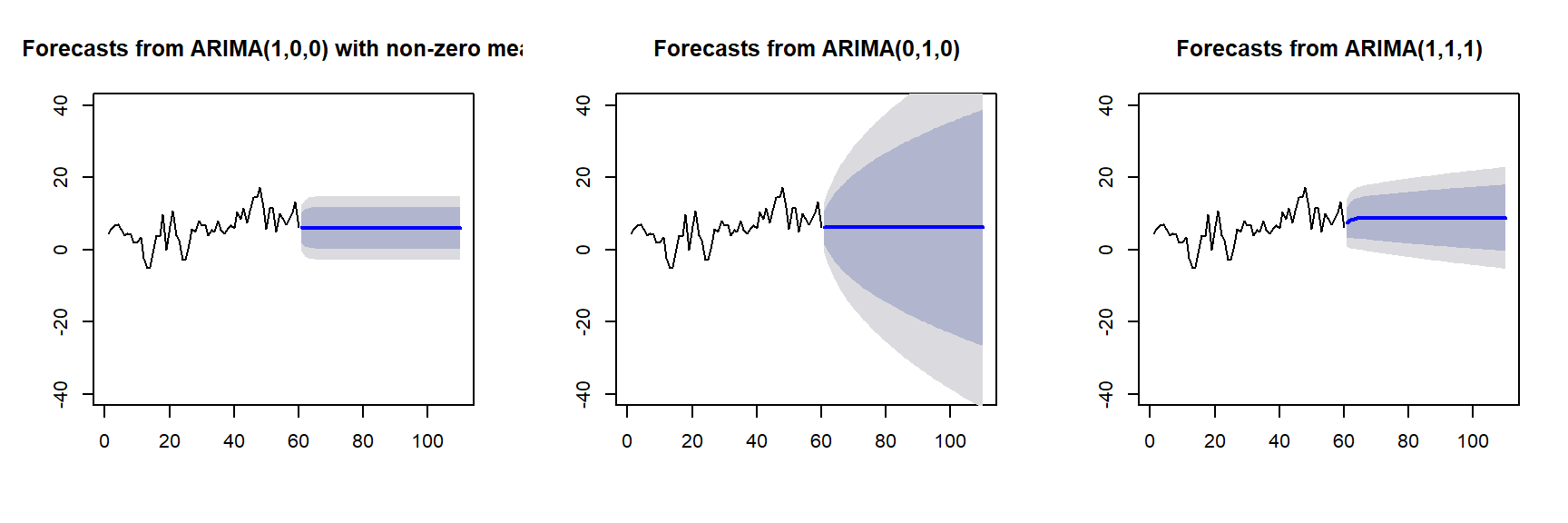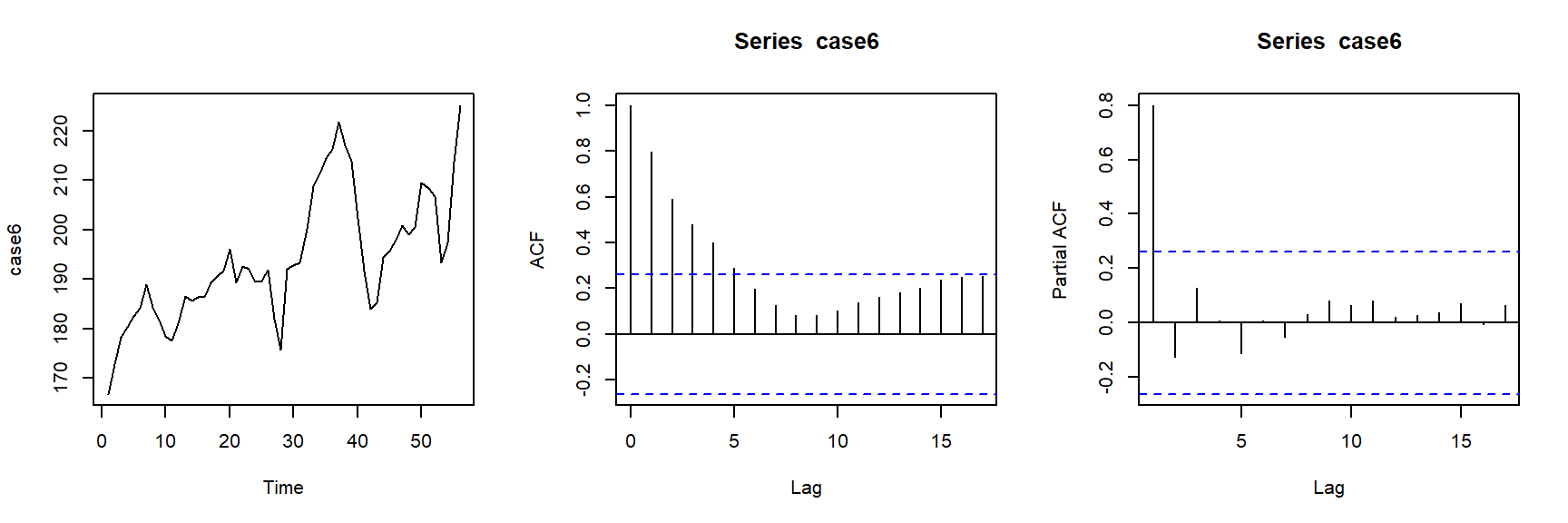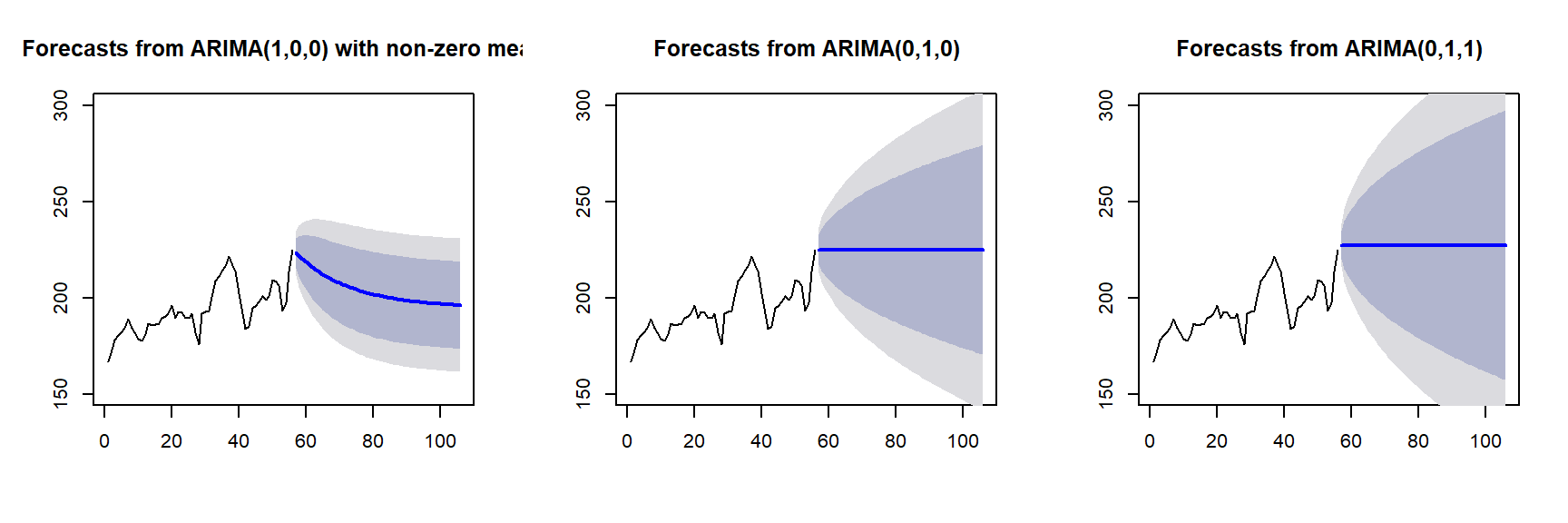R packages:

# Install necessary packages
list_packages <- c("forecast", "readxl", "stargazer", "fpp",
"fpp2", "scales", "quantmod", "urca",
"vars", "tseries", "ggplot2", "dplyr")
new_packages <- list_packages[!(list_packages %in% installed.packages()[,"Package"])]
if(length(new_packages)) install.packages(new_packages)

lapply(list_packages, require, character.only = TRUE)

# 1 Forecast Notations

After fitting models, the next step is to provide forecasting.

Before we introduce the forecast formula, let’s go over some notations.

$$z_n$$: last observation, $$z_1,...,z_n$$ are observed

$$t=n$$: forecast origin

$$z_{n+l}$$: value of the time series at lead time $$l$$, not observed

$$\hat{z}_{n}(l)$$: forecast of $$z_{n+l}$$ at forecast origin $$n$$.

$$\mathbb{E}_n[\cdot]$$: conditional expectation given all observations up to forecast origin $$n$$ (including forecast origin $$n$$), i.e., $$\mathbb{E}[\cdot|z_n,z_{n-1}...]$$.

Definition Best forecast of $$z_{n+l}$$ is the forecast $$\hat{z}_{n}(l)$$ which minimizes the expected mean square error, $$\mathbb{E}_n[(Z_{n+l} - \hat{z}_{n}(l))^2]$$.

Based on such a definition, we can prove that $$\mathbb{E}_n[Z_{n+l}]$$ is the best forecast of $$z_{n+l}$$, i.e., $$\mathbb{E}_n[Z_{n+l}]=\mathbb{E}[Z_{n+l}|z_n,z_{n-1}...]$$ is the best forecast.

So from now on, we let our forecast to be $$\hat{z}_{n}(l) = \mathbb{E}_n[Z_{n+l}]=\mathbb{E}[Z_{n+l}|z_n,z_{n-1}...]$$

# 2 Forecast by ARIMA Models

## 2.1 AR(1)

\begin{align*} \tilde{Z}_t &= \phi_1 \tilde{Z}_{t-1} + a_t\\ Z_{n+1} &= \mu + \phi_1 (Z_{n} - \mu ) + a_{n+1} \end{align*} Observe $$z_1, ..., z_n$$. Our forecast is \begin{align*} \hat{z}_{n}(1) &= \mathbb{E}_n [ Z_{n+1} ]\\ & = \mu + \phi_1 (\mathbb{E}_n [Z_n] - \mu ) + \mathbb{E}_n[a_{n+1}]\\ & = \mu + \phi_1 (z_n - \mu ) + 0 \end{align*}

By assumption $$\mathbb{E}_n[a_{n+1}]=0$$, because $$a_{n+1}$$ takes place after $$z_n, z_{n-1},...$$.

\begin{align*} \hat{z}_{n}(1) &= \mu + \phi_1 (z_n - \mu ) \end{align*}

Since $$\mu$$ and $$\phi_1$$ are unknown, so to forecast, we replace parameters by estimates, $$\hat{\mu}$$ and $$\hat{\phi}_1$$ and our final forecast is

\begin{align*} \hat{z}_{n}(1) &= \hat{\mu} + \hat{\phi}_1 (z_n - \hat{\mu} ) \end{align*}

We can see that \begin{align*} \hat{z}_{n}(2) &= \mathbb{E}_n [ Z_{n+2} ]\\ & = \mu + \phi_1 (\mathbb{E}_n [Z_{n+1}] - \mu ) + \mathbb{E}_n[a_{n+2}]\\ & = \mu + \phi_1 (\hat{z}_{n}(1) - \mu )\\ &= \mu + \phi_1^2 (z_n - \mu ) \end{align*}

In general,

\begin{align*} \hat{z}_{n}(l) &= \mu + \phi_1^l (z_n - \mu ) \end{align*}

for $$l=1,2,...$$

Note that for stationary AR(1), $$\phi_1^l \to 0$$ when $$l \to \infty$$ because $$|\phi_1|<1$$.

## 2.2 MA(1)

$\tilde{Z}_t = a_t - \theta_1 a_{t-1}$

Case 1: $$l>1$$

$Z_{n+l} = \mu + a_{n+l} - \theta_1 a_{n+l-1}\\ \hat{z}_{n}(l) = \mathbb{E}_n(Z_{n+l}) = \mu + 0 - 0 = \mu$

Case 2: $$l=1$$

Since $$Z_{n+1} = \mu + a_{n+1} - \theta_1 a_{n}$$, we have $\hat{z}_{n}(1) = \mathbb{E}_n(Z_{n+1}) = \mu + 0 - \theta_1 \mathbb{E}_n(a_{n})$

Since $$a_n$$ depends on current and past observations $$z_t$$’s, $$\mathbb{E}_n(a_n)$$ is not 0 any more. Thus we estimate $$\mathbb{E}_n(a_n)$$ by $$\hat{a}_n = z_n - \hat{z}_n =$$ residual at time $$n$$.

Therefore,

$\hat{z}_{n}(1) = \mu + 0 - \theta_1 \hat{a}_n$ To get a actual forecast, we plug in $$\hat{\mu}$$ and $$\hat{\theta}_1$$.

## 2.3 General Forecast

A general forecast formula of ARIMA

Suppose $$Z_t \sim ARIMA(p,d,q)$$, which can be considered as $$Z_t \sim ARMA(p+d,q)$$ (nonstationary)

\begin{align*} Z_t &= C + \Phi_1 Z_{t-1} + ... + \Phi_{p+d} Z_{t-p-d} + a_t - \theta_1 a_{t-1} ... - \theta_q a_{t-q} \end{align*}

\begin{align*} Z_{n+l} &= C + \Phi_1 Z_{n+l-1} + ... + \Phi_{p+d} Z_{n+l-p-d} + a_t - \theta_1 a_{n+l-1} ... - \theta_q a_{n+l-q} \end{align*}

The general formula \begin{align*} \hat{z}_{n}(l) =& \mathbb{E}[Z_{n+l}|\mathcal{F}_n] \\ =& C + \Phi_1 \mathbb{E}[Z_{n+l-1}|\mathcal{F}_n] + ... + \Phi_{p+d} \mathbb{E}[Z_{n+l-p-d}|\mathcal{F}_n] + \\ &\mathbb{E}[a_{n+l}|\mathcal{F}_n] - \theta_1 \mathbb{E}[a_{n+l-1}|\mathcal{F}_n] - ... - \theta_q \mathbb{E}[a_{n+l-q}|\mathcal{F}_n] \end{align*}

\begin{align*} \mathbb{E}[Z_{s}|\mathcal{F}_n]=\begin{cases} z_s, \quad \quad \text{ if } s \leq n\\ \hat{z}_n(s-n), \quad \text{ if } s > n \end{cases} \end{align*}

\begin{align*} \mathbb{E}[a_{s}|\mathcal{F}_n]=\begin{cases} \hat{a}_s=z_s - \hat{z}_{s-1}(1), \quad \text{ if } s \leq n\\ 0, \quad \quad \text{ if } s >n \end{cases} \end{align*}

Define

\begin{align*} \hat{Z}_{n+l}=\begin{cases} \hat{z}_n(l), \quad \quad \text{ if } l \geq 1\\ z_{n+l}, \quad \text{ if } l < 0 \end{cases} \end{align*}

\begin{align*} [a_{s}]=\begin{cases} \hat{a}_s, \quad \text{ if } s \leq n\\ 0, \quad \quad \text{ if } s > n \end{cases} \end{align*}

Then $\hat{z}_n(l) = C + \phi_1 \hat{Z}_{n+l-1} + ... + \phi_{p+d} \hat{Z}_{n+l-p-d} - \theta_1 [a_{n+l-1}] - ... - \theta_q [a_{n+l-q}]$

# 3 Limiting Properties of Forecasts

## 3.1 Long Run Mean of Forecast from Stationary Time Series Models

Let’s use AR(1) as an example.

\begin{align*} \hat{z}_n(l)&=\mathbb{E}_n[Z_{n+l}]\\ &=\mathbb{E}_n[u + \phi_1 (Z_{n+l-1}-u) + a_{n+l}]\\ &=\mathbb{E}_n[u + \phi_1^2 (Z_{n+l-2}-u) + \phi_1 a_{n+l-1} + a_{n+l}]\\ & ...\\ &=\mathbb{E}_n[\mu + a_{n+l} + \phi_1 a_{n+l-1} + \phi_1^2 a_{n+l-2} ... ]\\ &=\mu + 0 ... + 0 + \phi_1^l a_n + \phi_1^{(l+1)} a_{n-1} ... \end{align*} Note that $$|\phi_1|<1$$ for stationary time series model. Therefore, $$\phi_1^l \to 0$$ as $$l \to \infty$$. Therefore, the limit of the forecast is

\begin{align*} \lim_{l \to \infty} \hat{z}_n(l) = \mu + \lim_{l \to \infty} (\phi_1^l \hat{a}_n + \phi_1^{(l+1)} \hat{a}_{n-1} ...) = \mu \end{align*}

Therefore, the forecast converges to the mean for the stationary time series model. This result holds for AR(1), and it is generally true for all stationary AR, MA, ARMA models.

## 3.2 Long Run Mean of Forecast from Nonstationary Time Series Models

The forecast for nonstationary time series model do no revert to a fixed mean.

Consider a random walk $$Z_t=Z_{t-1}+a_t$$, we have

\begin{align*} \hat{z}_n(l)&= \begin{cases} z_n, \quad l=1\\ \hat{z}_n(l-1), \quad \quad l > 1 \end{cases} \end{align*}

So, we combine the cases and have

$\hat{z}_n(l) = z_n \text{ for }l \geq 1$

Thus, different realization of the same random walk model will result in forecasts converge to different values.

On the other hand, different realizations of the same stationary time series model will always produce the forecasts which converge to the same constant, $$\mu$$.

## 3.3 Long Run Variance of Forecast for Stationary and Nonstationary Models

Next, consider the limit of the variance of the forecast error of a stationary time series model. Let’s take AR(1) as an example.

$\lim_{l \to \infty} \text{Var}(Z_{n+l} - \hat{z}_{n}(l))\\ =\lim_{l \to \infty} \sigma_a^2(1+\phi_1^2+(\phi_1^2)^2+(\phi_1^{l-1})^2+...)\\ =\sigma_a^2 \sum_{l=0}^{\infty} (\phi_1^2)^l$

Thus the prediction “bands”about the forecast profile become parallel lines for large lead time ($$l \to \infty$$).

In fact, for AR(1), we can show

$\text{Var}(Z_{n+l} - \hat{z}_{n}(l)) = \sigma_a^2(1 + \phi_1^2 + \phi_1^4+\phi_1^{2(l-1)})$

On the other hand, consider the limit of the variance of the forecast error for a nonstationary time series, for example, random walk.

$(1-B)Z_t = a_t\\ Z_t = (1-B)^{-1} a_t\\ Z_t = (1+B+B^2+B^3...) a_t\\ Z_t = a_t + a_{t-1} + a_{t-2} + ...\\$ Therefore, the variance becomes

$\text{Var}(Z_{n+l} - \hat{z}_n(l)) = \sigma^2_a \sum_{j=0}^{l-1} 1 = \sigma^2_a * l$ So the variance $$\text{Var}(Z_{n+l} - \hat{z}_n(l))$$ tends to infinity when $$l \to \infty$$, so the prediction band expands.

# 4 Examples

case1=as.ts(scan("case1.txt"))
case1
## Time Series:
## Start = 1
## End = 60
## Frequency = 1
##    4.4  5.8  6.7  7.1  5.7  4.1  4.6  4.3  2.0  2.2  3.6 -2.2 -5.1 -4.9
##   0.1  4.1  3.8  9.9  0.0  6.5 10.8  4.1  2.7 -2.9 -2.9  1.5  5.7  5.0
##   7.9  6.8  7.1  4.1  5.5  5.1  8.0  5.6  4.5  6.1  6.7  6.1 10.6  8.6
##  11.6  7.6 10.9 14.6 14.5 17.4 11.7  5.8 11.5 11.7  5.0 10.0  8.9  7.1
##   8.3 10.2 13.3  6.2
par(mfrow=c(1,3))
plot(case1)
acf(case1)
pacf(case1)It seems fitting a stationary model would be sufficient.

fit <- arima(case1,order=c(1,0,0))
fit
##
## Call:
## arima(x = case1, order = c(1, 0, 0))
##
## Coefficients:
##          ar1  intercept
##       0.6803     6.0423
## s.e.  0.0914     1.2870
##
## sigma^2 estimated as 10.88:  log likelihood = -157.04,  aic = 320.08

However, the ADF test shows conflicting results.

adf.test(case1,k=1)
##
##  Augmented Dickey-Fuller Test
##
## data:  case1
## Dickey-Fuller = -3.5868, Lag order = 1, p-value = 0.04192
## alternative hypothesis: stationary
adf.test(case1,k=2)
##
##  Augmented Dickey-Fuller Test
##
## data:  case1
## Dickey-Fuller = -3.2623, Lag order = 2, p-value = 0.08614
## alternative hypothesis: stationary

So we decide to fit a nonstationary model again, and use auto.arima to fit a third model. We compare their forecast results.

par(mfrow=c(1,3))
plot(forecast(fit,h=50),ylim=c(-40,40))
fit2 <- arima(case1,order=c(0,1,0))
fit2
##
## Call:
## arima(x = case1, order = c(0, 1, 0))
##
##
## sigma^2 estimated as 13.07:  log likelihood = -159.53,  aic = 321.07
plot(forecast(fit2,h=50),ylim=c(-40,40))
fitauto <- auto.arima(case1)
fitauto
## Series: case1
## ARIMA(1,1,1)
##
## Coefficients:
##          ar1      ma1
##       0.5017  -0.8767
## s.e.  0.1643   0.0918
##
## sigma^2 estimated as 11.34:  log likelihood=-154.63
## AIC=315.26   AICc=315.7   BIC=321.5
plot(forecast(fitauto,h=50),ylim=c(-40,40))Case 6: AT&T stock

We repeat similar analysis as above for this AT&T stock data.

case6=as.ts(scan("case5.txt"))
case6
## Time Series:
## Start = 1
## End = 56
## Frequency = 1
##   166.8 172.8 178.3 180.3 182.6 184.2 188.9 184.4 181.7 178.5 177.6
##  181.0 186.5 185.7 186.4 186.3 189.3 190.6 191.7 196.1 189.3 192.6
##  192.1 189.4 189.7 191.9 182.0 175.7 192.0 192.8 193.3 200.2 208.8
##  211.4 214.4 216.3 221.8 217.1 214.0 202.4 191.7 183.9 185.2 194.5
##  195.8 198.0 200.9 199.0 200.6 209.5 208.4 206.7 193.3 197.3 213.7
##  225.1
par(mfrow=c(1,3))
plot(case6)
acf(case6)
pacf(case6)adf.test(case6)
##
##  Augmented Dickey-Fuller Test
##
## data:  case6
## Dickey-Fuller = -3.051, Lag order = 3, p-value = 0.1508
## alternative hypothesis: stationary
par(mfrow=c(1,3))
fit <- arima(case6,order=c(1,0,0))
fit
##
## Call:
## arima(x = case6, order = c(1, 0, 0))
##
## Coefficients:
##          ar1  intercept
##       0.9416   194.6908
## s.e.  0.0530    10.8988
##
## sigma^2 estimated as 35.64:  log likelihood = -180.61,  aic = 367.21
plot(forecast(fit,h=50),ylim=c(150,300))
fit2 <- arima(case6,order=c(0,1,0))
fit2
##
## Call:
## arima(x = case6, order = c(0, 1, 0))
##
##
## sigma^2 estimated as 36.12:  log likelihood = -176.68,  aic = 355.36
plot(forecast(fit2,h=50),ylim=c(150,300))
fitauto <- auto.arima(case6)
fitauto
## Series: case6
## ARIMA(0,1,1)
##
## Coefficients:
##          ma1
##       0.3589
## s.e.  0.1245
##
## sigma^2 estimated as 32.75:  log likelihood=-173.55
## AIC=351.1   AICc=351.33   BIC=355.11
plot(forecast(fitauto,h=50),ylim=c(150,300))